# Parameter Learning and the Intuition Behind Gradient Descent

In our previous article about the intuition behind the cost function and the process of "learning" in machine learning, we learned that machines try to optimize the cost function by trying out various values of parameters. Whichever set of parameters hits the minima of the cost function, the machine learns them and achieves intelligence.

But there is still a catch! If a machine has to try all possible combinations of parameters,

What are the chances that Machine will find those parameters for which the cost will be minimum?

Let's take the scenario of two parameters learning and plot the contour plot of the cost function with respect to the two parameters θ0 and θ1. We know that the cost function at the pink star will be the minima, and the corresponding values of parameters θ0 and θ1 will be learned by the machine.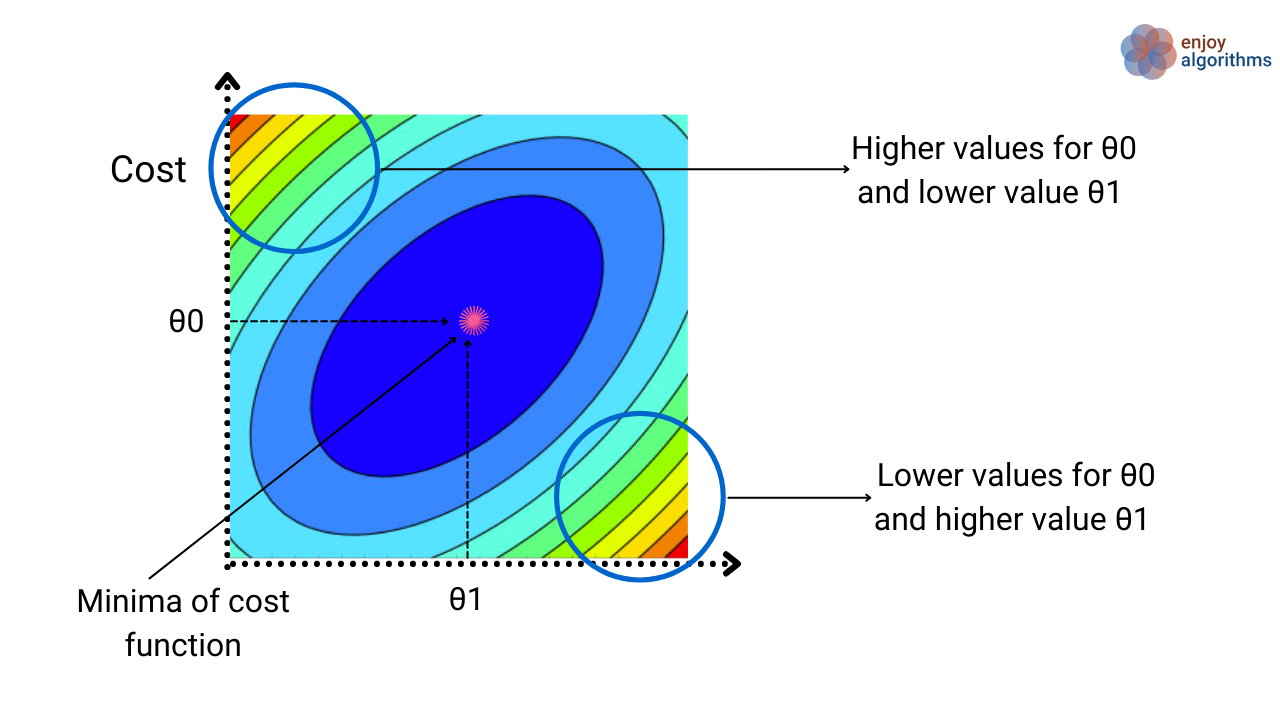To learn the optimal values of these parameters, machines randomly try combinations of (θ0, θ1). But if we ask our machines every time to take random values of these parameters, then machines can choose all the combinations of θ0 and θ1 from either of the two circular regions shown in the above image. It would take nearly infinite time to determine which (θ0, θ1) will take us to the pink star. We don't have that much amount of computing power to do these computations for infinite time.

To save us from this distress position, we need to take help from some optimization algorithms. Algorithms that can guide our Machines to take sensible steps and speed up finding optimal values of parameters.To understand this optimization thoroughly, let's define one cost problem with respect to the parameters and see the optimization steps.

In the equations below, suppose the cost function with respect to all the parameters that we need to learn is represented as J(θ0, θ1, …, θn). Our objective is to minimize this cost function with respect to the parameters.To keep things simple and easily visualizable, let's select only two parameters.#### Outline of Optimization Algorithm

The primary objective is already defined as optimizing the cost, and for that, any optimization algorithm will follow the standard outline, which is:

• Step 1: Start with some random values of parameters θ0, θ1.
• Step 2: Change the values of parameters θ0, θ1 such that J(θ0, θ1) becomes minimum.

#### Understanding this outline

In the image below, let's take a basic 3D plot of Cost function vs. parameters θ0, θ1 in the image below. If we notice, there can be multiple local minimum values. Following the basic outline of optimization algorithms, we randomly selected θ0 = 0 and θ1 = 0. We are at the "First initialization of parameters" point in the image below for this selection.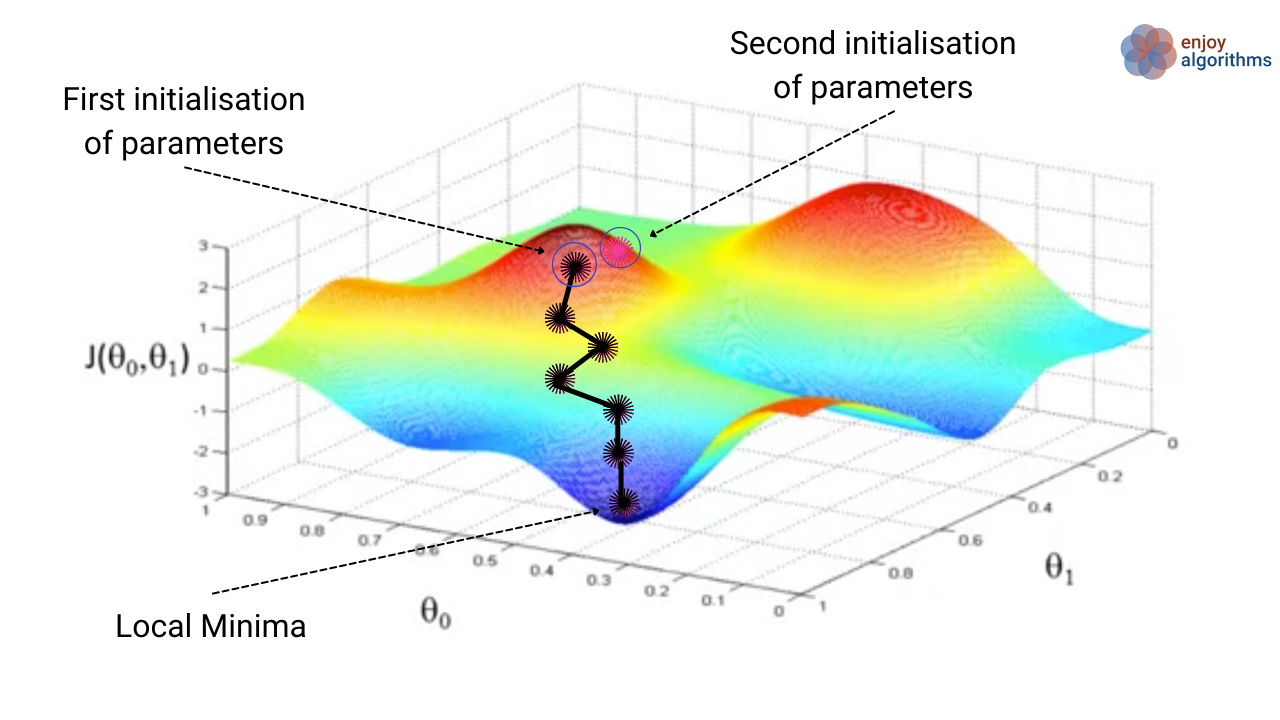If we slowly change the values of parameters in the direction in which the cost decreases, we might achieve the local minima position 1. At the same time, if we select some different initialization of parameters, let's say θ0 = 0.5 and θ1 = 0.5, we might end up finding some other local minima (local minima 2).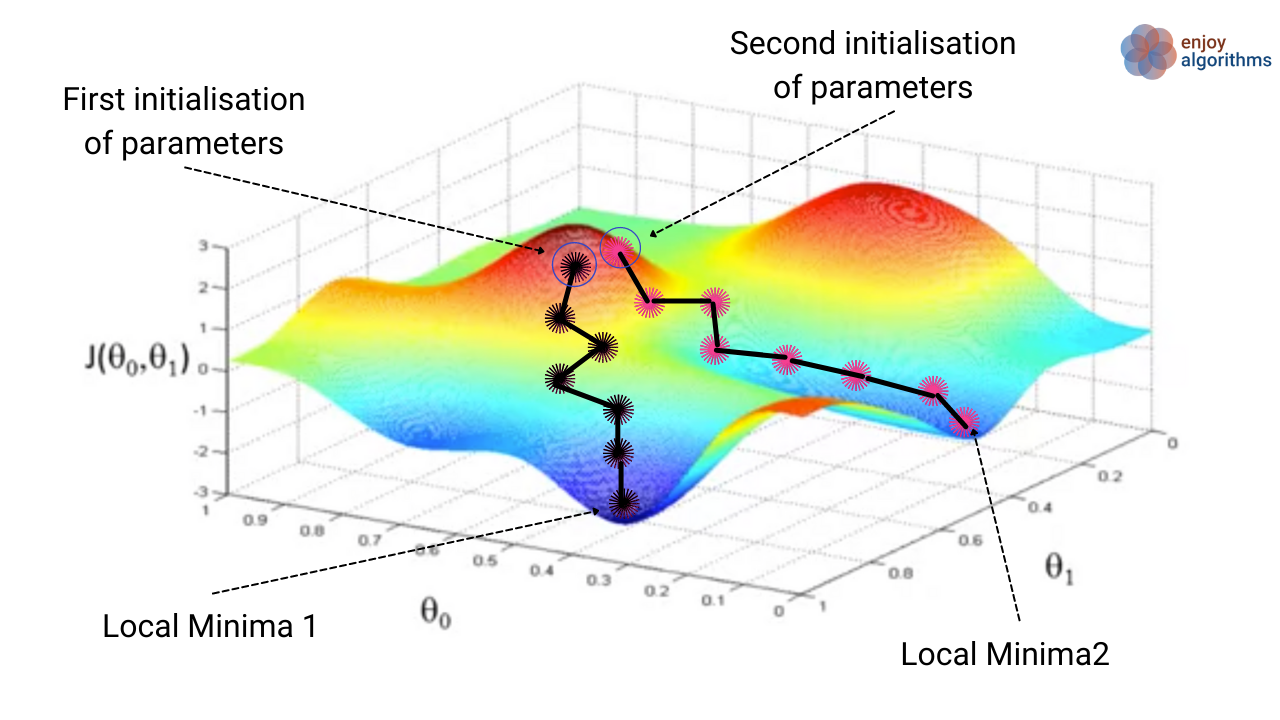We might be thinking, which one is correct then?

That's a valid question, but we must have understood that the initial selection of parameter values will significantly affect finding the minima. If local minima 1 is lesser than local minima 2, then the initial parameter choice with θ0 = 0 and θ1 = 0 is more accurate than other. Various optimization algorithm tries to make sure that we reach the global minimum value while finding the minimum of the cost function.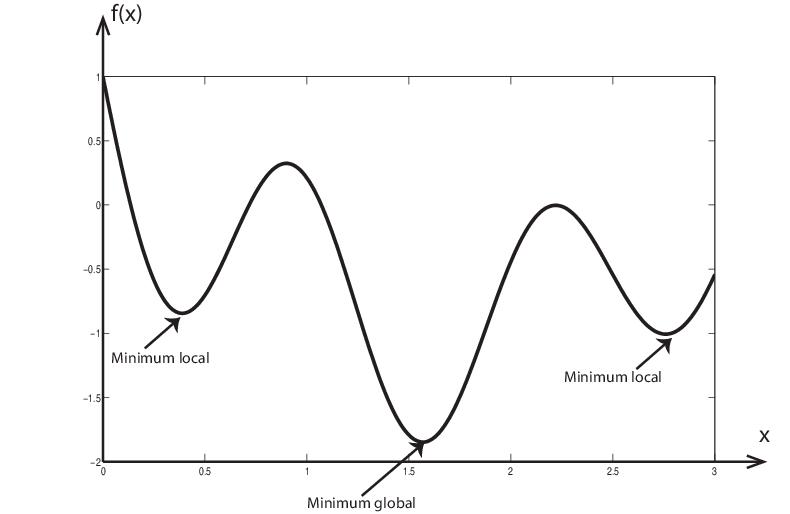Gradient Descent is one of the most basic and reasonably famous methods to optimize cost in machine learning among all the optimization algorithm. Let's learn this algorithm in greater detail.

Let's have a look at the pseudo-code for this algorithm,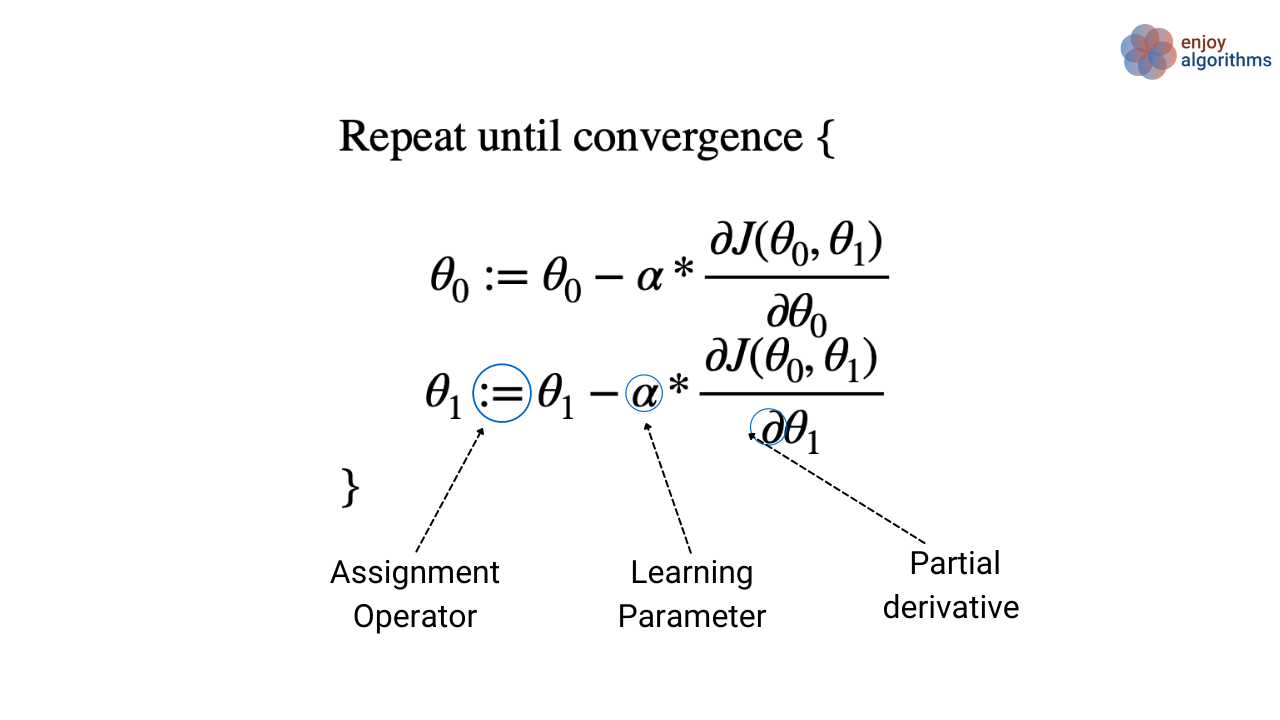#### Key terms in the above equation

:= is used to assign the Right-hand side value to the Left-hand side value. If we are familiar with the programming, then ":=" is similar to "=" as there also we assign RHS value to the LHS variable. Writing a = a + 1 in programming is identical to writing a := a+1 in the common description as a can never be equal to a + 1.

Learning Parameter (α): This Parameter controls the size of steps we take to update the Parameter.

Partial derivative: In the above equation, the cost function J varies with respect to two parameters, θ0 and θ1, simultaneously. Partially derivating J with respect to one Parameter means derivating the function J by considering J as a function of that Parameter only and considering another parameter constant.

In simple terms, we are updating the values of the parameters iteratively until the cost reaches a minimum value. But this update is also tricky. If we implement gradient descent like what is shown on the left side of the image below, it will be a wrong implementation. Can you guess why?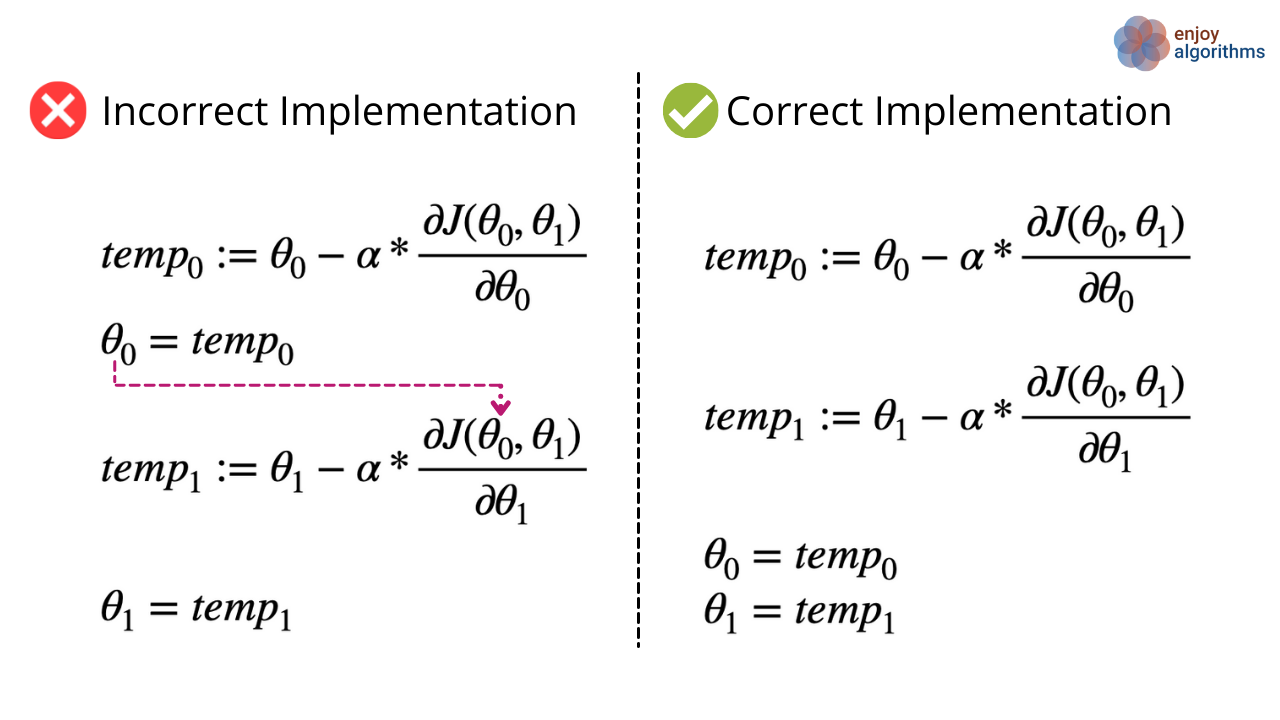The pink line is the reason. In the update of θ1, the value of θ0 will be used as the updated one, which is not right for gradient descent. In the gradient descent algorithm, we first calculate what new parameters value and then assign the new values to the previous parameters in the last simultaneously.

But why this type of update of parameters?

To answer this, let's have a look at the intuition behind this algorithm.

#### The intuition behind the Gradient Descent algorithm

Let's take the example of one-parameter learning (Just θ1) and see how this unique updating fashion helps find the optimal parameter values.

Suppose cost is varying with parameter theta, as shown in the image below.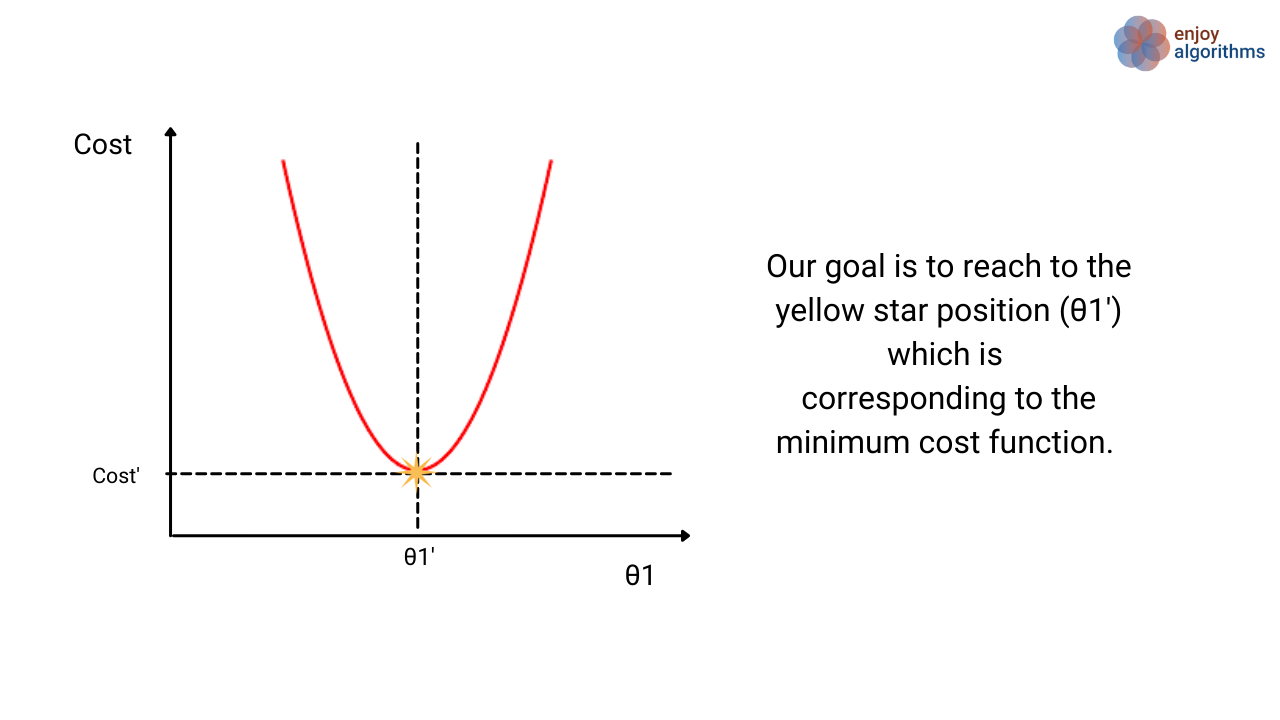From our understanding of the outline of optimization algorithms, we first select the initial value of the Parameter. In this case of a single parameter, that initial guess can be anywhere but either in the two regions of the right side of the yellow star or the left side of the yellow star unless we are lucky enough to hit the minima in our first guess.

So, our job is done if our optimization algorithm somehow shows that we have to decrease/increase the θ1 value to reach the yellow star position. Right?

In gradient descent, we do the same by using the property of the derivative. In the region right side to the yellow star, the derivative or slope will be positive, and in the region left side to the yellow star, the derivative would be negative. At the yellow star, the derivative will be zero as well.

Our learning parameter α is going to be always a positive constant for gradient descent. The sign of the derivative will tell us whether we are increasing or decreasing the values of parameter θ1.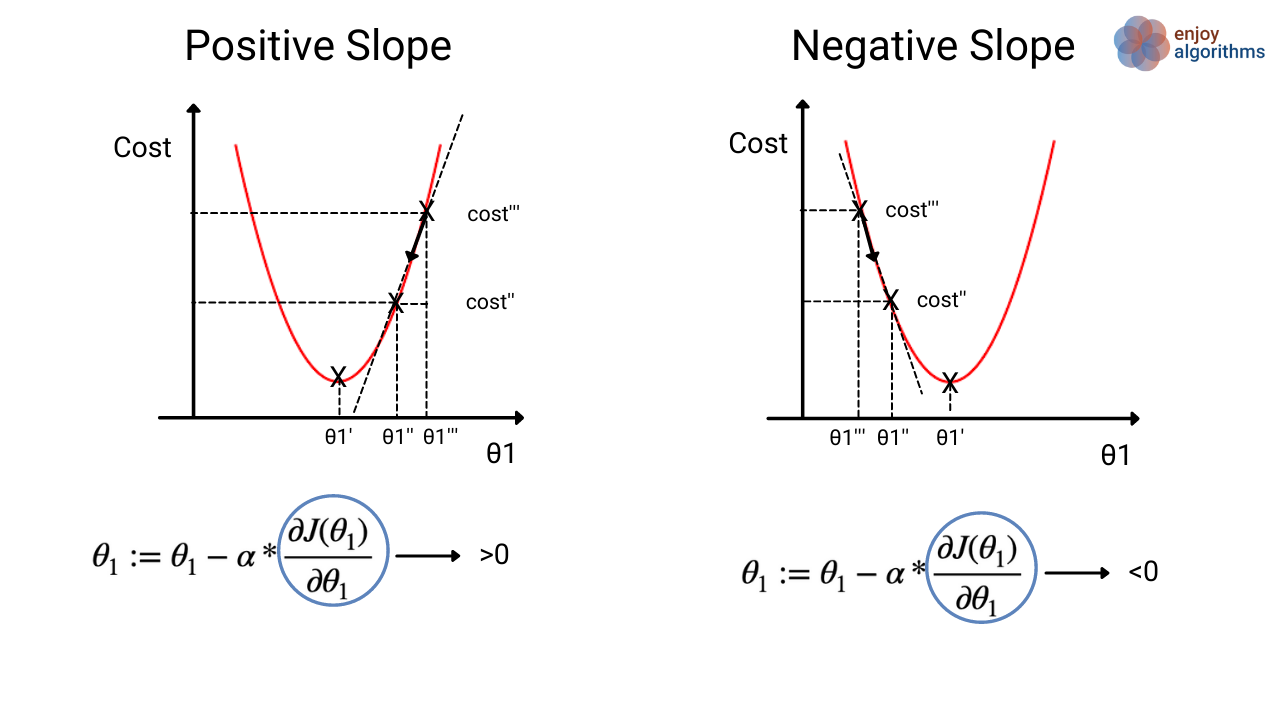But then another question should be raised, what is the need for α? Indeed a valid question because the derivative itself can decide whether we need to increase or decrease the value of θ1.

Even if we decide to increase or decrease, it could also take infinite time to reach the yellow star as we will be updating the parameters very slowly. To help in this situation, the parameter α tells us whether we need to change the Parameter's value by a greater margin or smaller margin based on the position of θ1.

#### There are two conditions:

• Condition 1: If we will select a very small value for α, then the gradient descent algorithm will become very slow and may take a very large time to achieve the minimum cost function.
• Condition 2: If we select a very large value for α, then the gradient descent algorithm will overshoot the minimum values or might not reach the minimum of the cost function.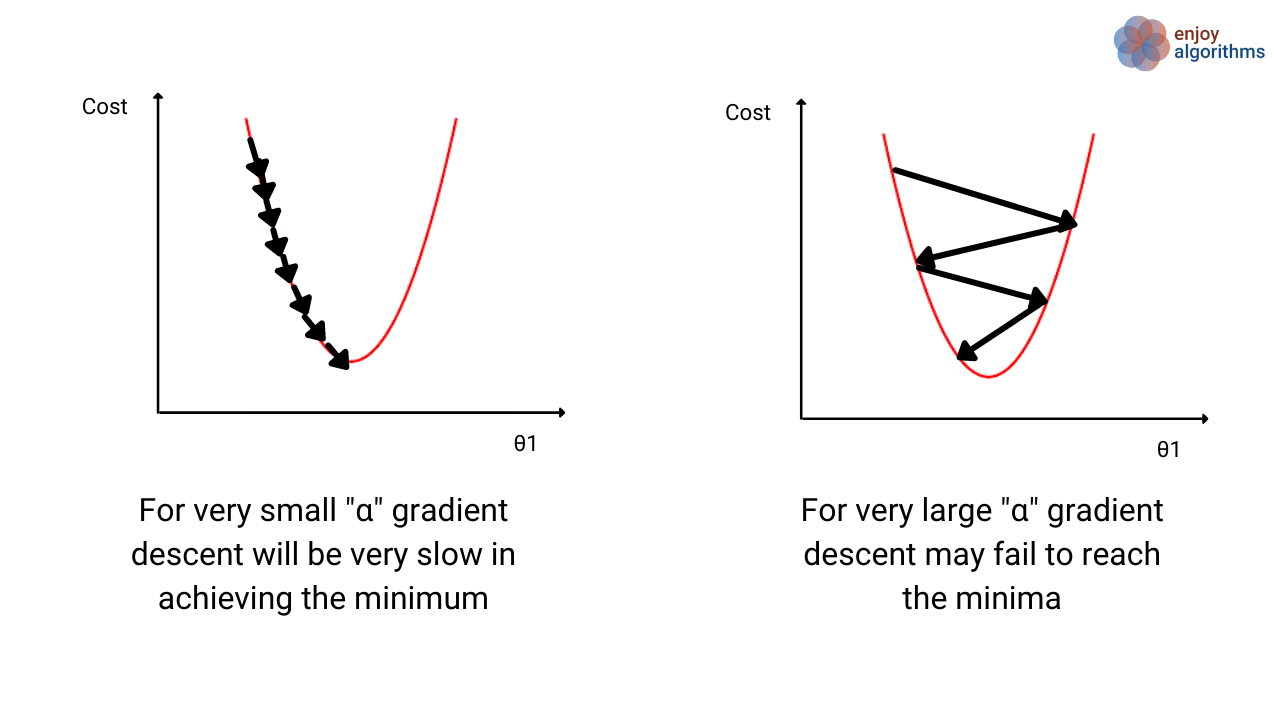We must be thinking that,

Should we change the value of α in the process like choosing a larger value when θ1 is far from θ1' and choosing a smaller value when θ1 is near to θ1'?

In gradient descent algorithms, we keep the value of α constant throughout the training process. Based on the derivative values, the algorithm starts taking smaller steps when θ1 nears θ1'.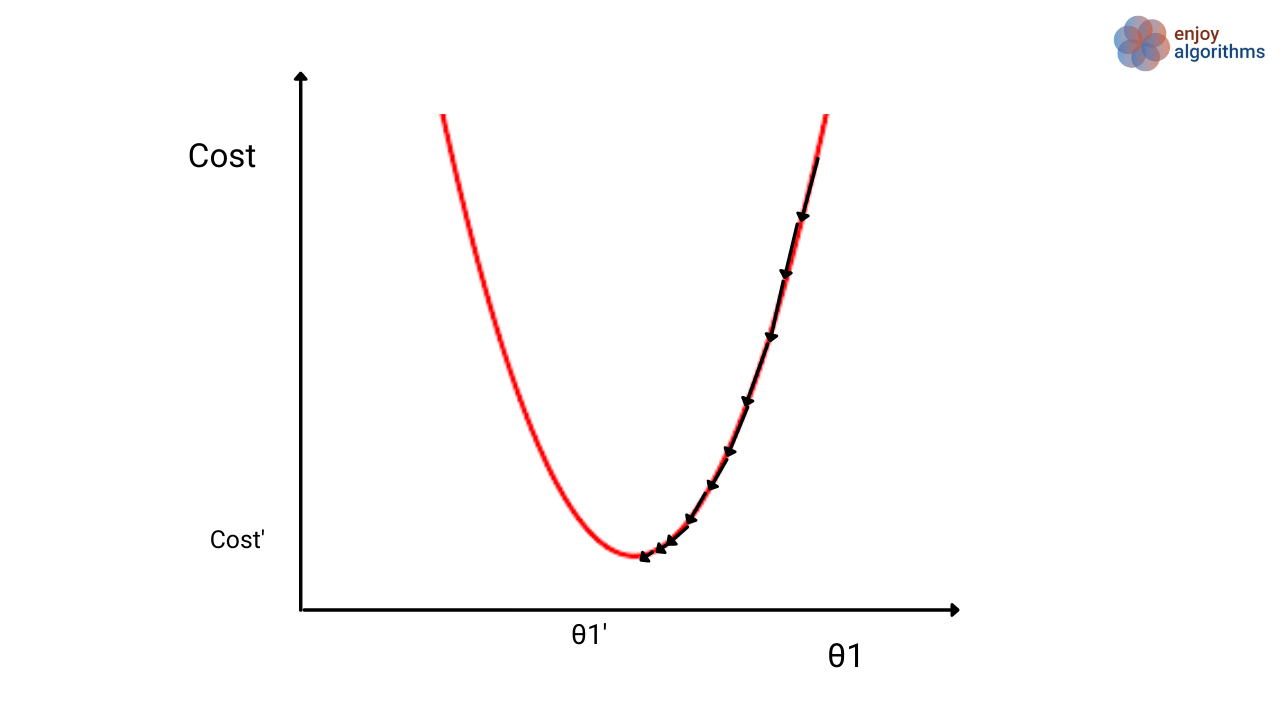Some algorithms change the values of α based on the parameter values, but we are not discussing them here. These algorithms do not guarantee to make us reach the global minima but speed up finding the local minima and possibly the global minima.

#### Possible Interview Questions

The topic of gradient descent in Machine Learning is considered being fundamental. Every interviewer expects you to know about it. In data scientist interviews, it is quite probable to be asked on gradient descent algorithm. Possible interview questions on this topic could be,

1. What is the role of gradient descent in making machines learn?
2. Questions on the correct and incorrect ways of implementing the gradient descent algorithm.
3. How do we decide the value of the learning parameter?
4. Will gradient descent always help in finding the global minimum?
5. Do we need to change the value of the learning parameter in gradient descent?

#### Conclusion

In this article, we have discussed one of the most basic cost optimization algorithms, gradient descent. We learned how this helps us find the right set of parameters for learning in machine learning. The effect of the learning parameter is also discussed with choosing the smaller and larger values for it. We hope you learned something new and enjoyed the article.

#### References

1. Andrew Ng Lectures

#### Enjoy Learning! Enjoy Optimizing! Enjoy Algorithms!

Subscribe to get well-designed content on data structures and algorithms, machine learning, system design, oops, and mathematics. enjoy learning!

### We Welcome Doubts and Feedback!